×#### Thank you for registering.

One of our academic counsellors will contact you within 1 working day.

Click to Chat

1800-1023-196

+91-120-4616500

CART 0

• 0

MY CART (5)

Use Coupon: CART20 and get 20% off on all online Study Material

ITEM
DETAILS
MRP
DISCOUNT
FINAL PRICE
Total Price: Rs.

There are no items in this cart.
Continue Shopping• Complete JEE Main/Advanced Course and Test Series
• OFFERED PRICE: Rs. 15,900
• View Details

```Chapter 14: Quadrilaterals Exercise – 14.4

Question: 1

In ΔABC, D, E and F are, respectively the mid points of BC, CA and AB. If the lengths of sides AB, BC and CA are 7cm, 8 cm and 9 cm, respectively, find the perimeter of ΔDEF.

Solution:

Given that,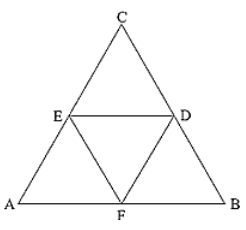AB = 7 cm, BC = 8 cm, AC = 9 cm

In ∆ABC,

F and E are the mid points of AB and AC.

∴ EF = 1/2 BC

Similarly

DF = 1/2 AC and DE = 1/2 AB

Perimeter of ∆DEF = DE + EF + DF

= (1/2) AB + (1/2) BC + (1/2) AC

= 1/2 ∗7 + 1/2 ∗8 + 1/2 ∗9

= 3.5 + 4 + 4.5

= 1/2 cm

Perimeter of ΔDEF = 1/2 cm

Question: 2

In a ΔABC, ∠A = 50°, ∠B = 60° and ∠C = 70°. Find the measures of the angles of the triangle formed by joining the mid-points of the sides of this triangle.

Solution:

In ΔABC,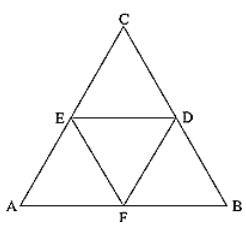D and E are mid points of AB and BC.

By Mid point theorem,

DE ∥ AC, DE = (1/2) AC

F is the midpoint of AC

Then, DE = (1/2) AC = CF

DE ∥ AC, DE = CF

Hence DECF is a parallelogram.

∴ ∠C = ∠D = 70°        [Opposite sides of a parallelogram]

Similarly

BEFD is a parallelogram, ∠B = ∠F = 60°

ADEF is a parallelogram, ∠A = ∠E = 50°

∴ Angles of ΔDEF are

∠D = 70°, ∠E = 50°, ∠F = 60°

Question: 3

In a triangle, P, Q and R are the mid points of sides BC, CA and AB respectively. If AC = 21cm, BC = 29 cm and AB = 30 cm, find the perimeter of the quadrilateral ARPQ.

Solution:

In ΔABC,

R and P are mid points of AB and BC

RP ∥ AC, RP = (1/2) AC [By Midpoint Theorem]In a quadrilateral,

[A pair of side is parallel and equal]

RP ∥ AQ, RP = AQ

Therefore, RPQA is a parallelogram

⇒ AR = (1/2) AB = 1/2∗30 = 15 cm

AR = QP = 15 cm                 [Opposite sides are equal]

⇒ RP = (1/2) AC =1/2 ∗21 = 10.5 cm

RP = AQ = 10.5 cm             [Opposite sides are equal]

Now,

Perimeter of ARPQ = AR + QP + RP + AQ

= 15 + 15 + 10.5 + 10.5

= 51 cm

Question: 4

In a ΔABC median AD is produced to x such that AD = DX. Prove that ABXC is a parallelogram.

Solution: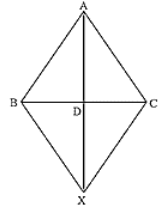In a quadrilateral ABXC, we have

BD = DC [Given]

So, diagonals AX and BC bisect each other.

Therefore, ABXC is a parallelogram.

Question: 5

In a ΔABC, E and F are the mid-points of AC and AB respectively. The altitude AP to BC intersects FE at Q. Prove that AQ = QP.

Solution: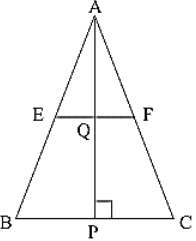In a ΔABC

E and F are mid points of AB and AC

∴  EF ∥ FE, (1/2) BC = FE   [By midpoint theorem]

In ΔABP

F is the mid-point of AB and ∴ FQ ∥ BP    [∴ EF ∥ BP]

Therefore, Q is the mid-point of AP    [By mid-point theorem]

Hence, AQ = QP.

Question: 6

In a ΔABC, BM and CN are perpendiculars from B and C respectively on any line passing through A. If L is the mid-point of BC, prove that ML = NL.

Solution:Given that,

In ΔBLM and ΔCLN

∠BML = ∠CNL = 90°

BL = CL         [L is the mid-point of BC]

∠MLB = ∠NLC      [Vertically opposite angle]

∴  ΔBLM = ΔCLN

∴  LM = LN         [corresponding parts of congruent triangles]

Question: 7

In figure, Triangle ABC is a right angled triangle at B. Given that AB = 9 cm, AC = 15 cm and D, E are the mid-points of the sides AB and AC respectively, calculate

(i) The length of BC

Solution:

In ΔABC, ∠B = 900,

By using Pythagoras theoremAC2 = AB2 + BC2

⟹152 = 92 + BC2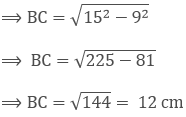In ΔABC,

D and E are mid-points of AB and AC

∴ DE ∥ BC, DE = (1/2) BC [By mid−point theorem]

AD = DB = AB/2 = 9/2 = 4.5 cm [∵ D is the mid−point of AB]]

= 1/2 ∗4.5∗6

= 13.5 cm2

Question: 8

In figure, M, N and P are mid-points of AB, AC and BC respectively. If MN = 3 cm, NP = 3.5 cm and MP = 2.5 cm, calculate BC, AB and AC.

Solution:

Given MN = 3 cm, NP = 3.5 cm and MP = 2.5 cm.

To find BC, AB and ACIn ΔABC

M and N are mid-points of AB and AC

∴ MN = (1/2) BC, MN ∥ BC   [By mid-point theorem]

⇒ 3 = (1/2) BC

⇒ 3∗2 = BC

⇒ BC = 6 cm

Similarly

AC = 2MP = 2 (2.5) = 5 cm

AB = 2 NP = 2 (3.5) = 7 cm

Question: 9

ABC is a triangle and through A, B, C lines are drawn parallel to BC, CA and AB respectively intersecting at P, Q and R. Prove that the perimeter of ΔPQR is double the perimeter of ΔABC.Solution:

Clearly ABCQ and ARBC are parallelograms.

Therefore, BC = AQ and BC = AR

⟹ AQ = AR

⟹ A is the mid-point of QR

Similarly B and C are the mid points of PR and PQ respectively.

∴ AB = (1/2) PQ, BC = (1/2) QR, CA = (1/2) PR

⟹ PQ = 2AB, QR = 2BC and PR = 2CA

⟹ PQ + QR + RP = 2 (AB + BC + CA)

⟹ Perimeter of ΔPQR = 2 (perimeter of ΔABC)

Question: 10

In figure, BE⊥ AC, AD is any line from A to BC intersecting BE in H. P, Q and R are respectively the mid-points of AH, AB and BC. Prove that ∠PQR = 90°Solution:

Given,

BE ⊥ AC and P, Q and R are respectively mid-point of AH, AB and BC.

To prove: ∠PQR = 90°

Proof: In ΔABC, Q and R are mid-points of AB and BC respectively.

∴ QR ∥ AC     ..... (i)

In ΔABH, Q and P are the mid-points of AB and AH respectively

∴ QP ∥ BH   ..... (ii)

But, BE⊥AC

Therefore, from equation (i) and equation (ii) we have,

QP⊥QR

⟹ ∠PQR = 90°

Hence Proved.

Question: 11

In figure, AB = AC and CP ∥ BA and AP is the bisector of exterior ∠CAD of ∆ABC. Prove that

(i) ∠PAC = ∠BCA.

(ii) ABCP is a parallelogram.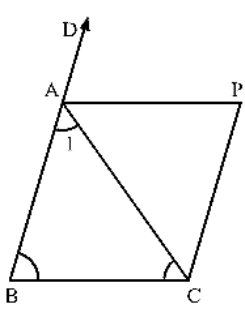Solution:

Given,

AB = AC and CD ∥ BA and AP is the bisector of exterior ∠CAD of ΔABC

To prove:

(i) ∠PAC = ∠BCA

(ii) ABCP is a parallelogram.

Proof:

(i) We have,

AB = AC

⟹ ∠ACB = ∠ABC      [Opposite angles of equal sides of triangle are equal]

Now, ∠CAD = ∠ABC + ∠ACB

⟹ 2∠PAC = 2∠ACB

⟹ ∠PAC = ∠ACB

(ii) Now,

∠PAC = ∠BCA

⟹ AP ∥ BC and CP ∥ BA     [Given]

Therefore, ABCP is a parallelogram.

Question: 12

ABCD is a kite having AB = AD and BC = CD. Prove that the figure found by joining the mid points of the sides, in order, is a rectangle.

Solution:

Given,

A kite ABCD having AB = AD and BC = CD. P, Q, R, S are the mid-points of sides AB, BC, CD and DA respectively. PQ, QR, RS and SP are joined.

To prove:

PQRS is a rectangle.Proof:

In ∆ABC, P and Q are the mid-points of AB and BC respectively.

∴ PQ ∥ AC and PQ = (1/2) AC        .... (i)

In ∆ADC, R and S are the mid-points of CD and AD respectively.

∴ RS ∥ AC and RS = (1/2) AC     .... (ii)

From (i) and (ii) we have

PQ ∥ RS and PQ = RS

Thus, in quadrilateral PQRS, a pair of opposite sides is equal and parallel. So, PQRS is a parallelogram. Now, we shall prove that one angle of parallelogram PQRS is a right angle.

⇒ AP = AS      ... (iii)         [∵ P and S are midpoints of AB and AD]

⇒ ∠1 = ∠2             .... (iv)

Now, in ΔPBQ and ΔSDR, we have

PB = SD              [∴ AD = AB ⇒ (1/2) AD = (1/2) AB]

BQ = DR            [Since PB = SD]

And PQ = SR     [Since, PQRS is a parallelogram]

So, by SSS criterion of congruence, we have

ΔPBQ ≅ ΔSDR

⇒∠3 = ∠4 [CPCT]

Now, ⇒ ∠3 + ∠SPQ + ∠2 = 180°

And ∠1 + ∠PSR + ∠4 = 180°

∴ ∠3 + ∠SPQ + ∠2 = ∠1 + ∠PSR + ∠4

⇒ ∠SPQ = ∠PSR               [∠1 = ∠2 and ∠3 = ∠4]

Now, transversal PS cuts parallel lines SR and PQ at S and P respectively.

∴ ∠SPQ + ∠PSR = 180°

⟹ 2∠SPQ = 180°

⟹ ∠SPQ = 90°              [∵ ∠PSR = ∠SPQ]

Thus, PQRS is a parallelogram such that ∠SPQ = 90°.

Hence, PQRS is a parallelogram.

Question: 13

Let ABC be an isosceles triangle in which AB = AC. If D, E, F be the mid points of the, sides BC, CA and AB respectively, show that the segment AD and EF bisect each other at right angles.

Solution:

Since D, E and F are mid-points of sides BC, CA and AB respectively.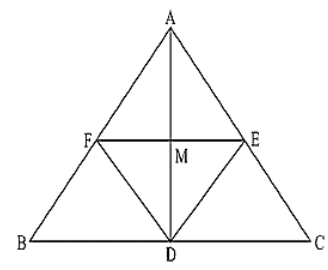∴  AB ∥ DE and AC ∥ DF

∴  AF ∥ DE and AE ∥ DF

ABDE is a parallelogram.

AF = DE and AE = DF

(1/2) AB = DE and (1/2) AC = DF

DE = DF             [Since, AB = AC]

AE = AF = DE = DF

ABDF is a rhombus.

⟹ AD and FE bisect each other at right angle.

Question: 14

ABC is a triangle. D is a point on AB such that AD = (1/4) AB and E is a point on AC such that AE = (1/4) AC. Prove that DE = (1/4) BC.

Solution:

Let P and Q be the mid-points of AB and AC respectively.Then PQ ∥ BC

PQ = (1/2) BC   .... (i)

In ΔAPQ, D and E are the mid-points of AP and AQ respectively.

(1/2) DE ∥ PQ, and DE = (1/2) PQ   .... (ii)

From (i) and (ii): DE = (1/2) PQ = (1/2) ((1/2) BC)

∴ DE = (1/2) BC

Hence proved.

Question: 15

In Figure, ABCD is a parallelogram in which P is the mid-point of DC and Q is a point on AC such that CQ = (1/2) AC. If PQ produced meets BC at R, prove that R is a mid-point of BC.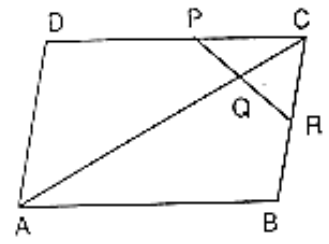Solution:

Join B and D.

Suppose AC and BD intersect at O.Then OC = (1/2) AC

Now,

CQ = (1/4) AC

⇒ CQ = 1/2((1/2) AC)

= (1/2) OC

In ΔDCO, P and Q are mid points of DC and OC respectively.

∴ PQ ∥ DO

Also in ΔCOB, Q is the mid-point of OC and QR ∥ OB

Therefore, R is the mid-point of BC.

Question: 16

In figure, ABCD and PQRC are rectangles and Q is the mid-point of AC. Prove that

(i) DP = PC

(ii) PR = (1/2) ACSolution:

(i) In ΔADC, Q is the mid-point of AC such that PQ∥AD

Therefore, P is the mid-point of DC.

⟹ DP = DC        [Using mid-point theorem]

(ii) Similarly, R is the mid-point of BC

∴  PR = (1/2) BD

PR = (1/2) AC   [Diagonal of rectangle are equal, BD = AC]

Question: 17

ABCD is a parallelogram; E and f are the mid-points of AB and CD respectively. GH is any line intersecting AD, EF and BC at G, P and H respectively. Prove that GP = PH.

Solution:

Since E and F are mid-points of AB and CD respectivelyAE = BE = (1/2) AB

And CF = DF = (1/2) CD

But, AB = CD

(1/2)AB = (1/2) CD

⟹ BE = CF

Also, BE ∥ CF [∴ AB ∥ CD]

Therefore, BEFC is a parallelogram

BC ∥ EF and BE = PH  .... (i)

Now, BC ∥ EF

⟹ AD ∥ EF         [∵ BC ∥ AD as ABCD is a parallelogram]

Therefore, AEFD is a parallelogram.

⟹ AE = GP

But E is the mid-point of AB.

So, AE = BF

Therefore, GP = PH.

Question: 18

BM and CN are perpendiculars to a line passing through the vertex A of triangle ABC. If L is the mid-point of BC, prove that LM = LN.

Solution:

To prove LM = LNDraw LS as perpendicular to line MN.

Therefore, the lines BM, LS and CN being the same perpendiculars on line MN are parallel to each other.

According to intercept theorem,

If there are three or more parallel lines and the intercepts made by them on a transversal are equal, then the corresponding intercepts on any other transversal are also equal.

In the figure, MB, LS and NC are three parallel lines and the two transversal lines are MN and BC.

We have, BL = LC   [As L is the given mid-point of BC]

Using the intercept theorem, we get

MS = SN  ..... (i)

Now in ΔMLS and ΔLSN

MS = SN using equation ... (i)

∠LSM = ∠LSN = 90° [LS ⊥ MN]

And SL = LS is common.

∴  ΔMLS ≅ ΔLSN    [SAS Congruency Theorem]

∴  LM = LN           [CPCT]

Question: 19

Show that, the line segments joining the mid-points of opposite sides of a quadrilateral bisects each other.

Solution:

Let ABCD is a quadrilateral in which P, Q, R and S are mid-points of sides AB, BC, CD and DA respectively.So, by using mid-point theorem we can say that

SP ∥ BD and SP = (1/2) BD          .... (i)

Similarly in ΔBCD

QR ∥ BD and QR = (1/2) BD          .... (ii)

From equations (i) and (ii), we have

SP ∥ QR and SP = QR

As in quadrilateral SPQR, one pair of opposite sides is equal and parallel to each other.

So, SPQR is a parallelogram since the diagonals of a parallelogram bisect each other.

Hence PR and QS bisect each other.

Question: 20

Fill in the blanks to make the following statements correct:

(i) The triangle formed by joining the mid-points of the sides of an isosceles triangle is ____________.

(ii) The triangle formed by joining the mid-points of the sides of a right triangle is ____________.

(iii) The figure formed by joining the mid-points of consecutive sides of a quadrilateral is ____________.

Solution:

(i) Isosceles

(ii) Right triangle

(iii) Parallelogram
```### Course Features

• 728 Video Lectures
• Revision Notes
• Previous Year Papers
• Mind Map
• Study Planner
• NCERT Solutions
• Discussion Forum
• Test paper with Video Solution